# 2 Interpreting the Periodic Table 3 Interpreting the

• Slides: 40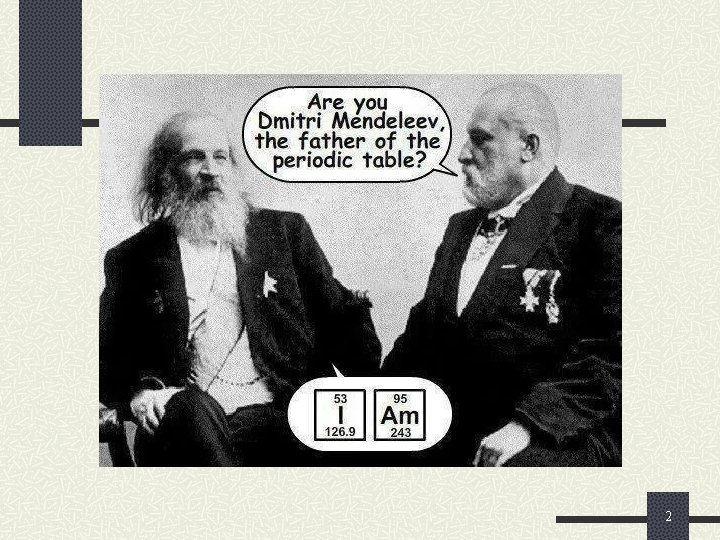2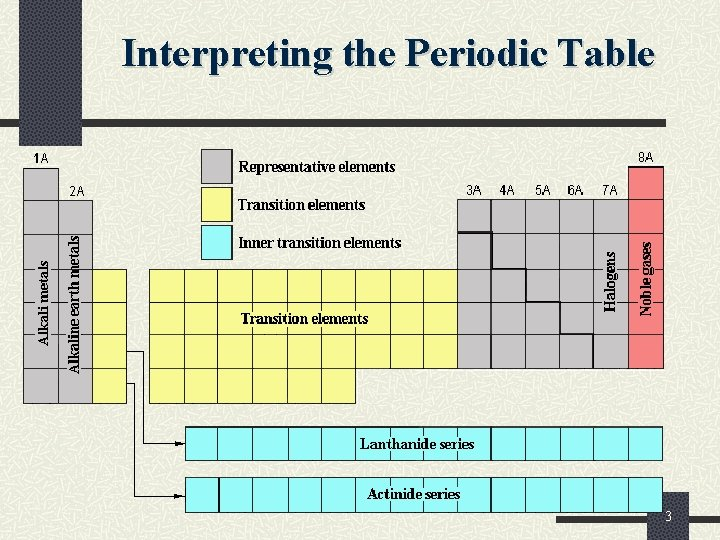Interpreting the Periodic Table 3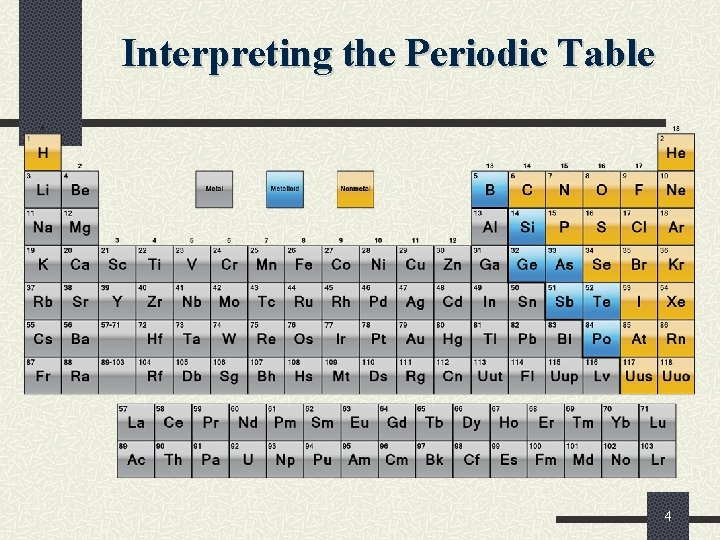Interpreting the Periodic Table 4General Properties of Metallic Elements: 1. Typically they have a shiny luster. 2. Relatively high density. 3. Malleable ( they can be hammered into thin sheets). 4. Ductile (they can be drawn into a wire). 5. Good conductors of electricity. 6. Reflect light and heat. 7. High melting and boiling points so they are solids at room temperature (except Hg). 8. Lose electron(s) forming positive ions (cations). 9. Combine with non-metals. 10. Do not readily combine with each other.Examples of Metals Potassium, K reacts with water and must be stored in kerosene Copper, Cu, is a relatively soft metal, and a very good electrical conductor. Zinc, Zn, is more stable than potassium Mercury, Hg, is the only metal that exists as a liquid at room temperature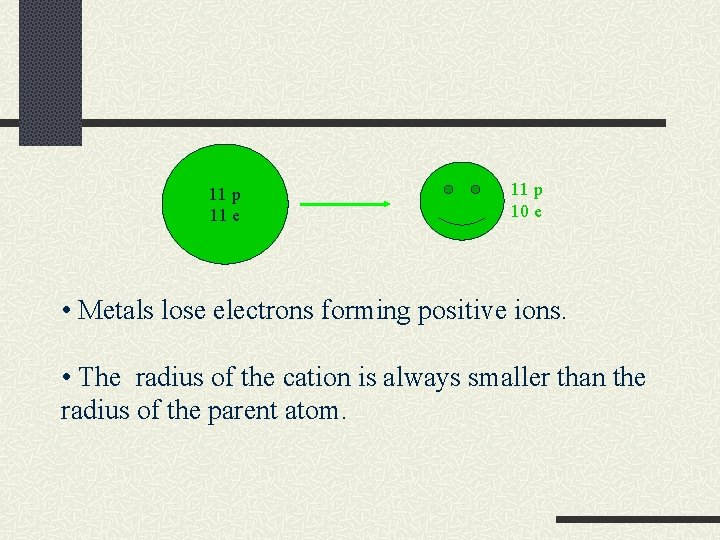11 p 11 e 11 p 10 e • Metals lose electrons forming positive ions. • The radius of the cation is always smaller than the radius of the parent atom.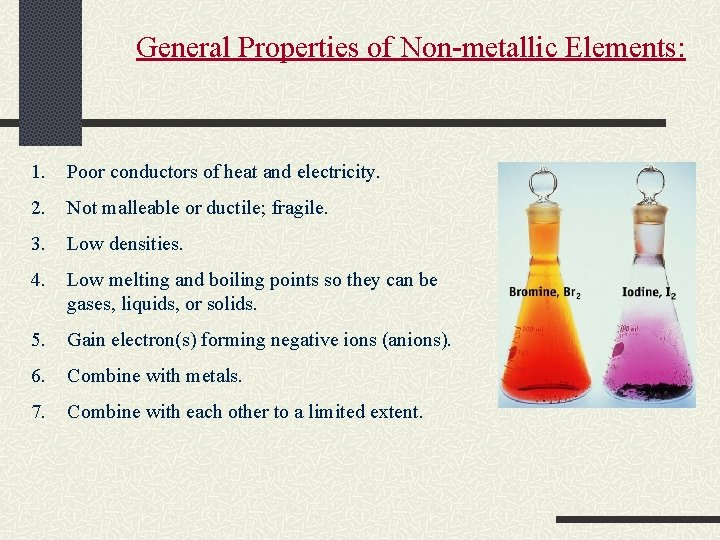General Properties of Non-metallic Elements: 1. Poor conductors of heat and electricity. 2. Not malleable or ductile; fragile. 3. Low densities. 4. Low melting and boiling points so they can be gases, liquids, or solids. 5. Gain electron(s) forming negative ions (anions). 6. Combine with metals. 7. Combine with each other to a limited extent.17 p 17 e 17 p 18 e • Non metals gain electrons forming negative ions. • The radius of the anion is always larger than the radius of the parent atom.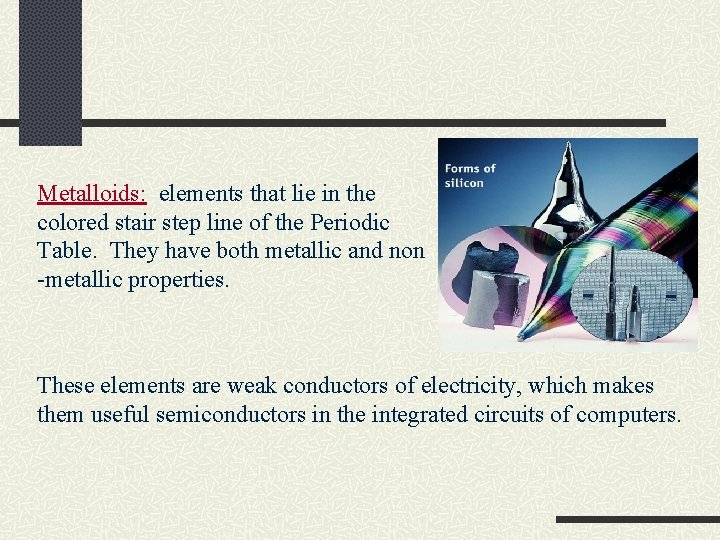Metalloids: elements that lie in the colored stair step line of the Periodic Table. They have both metallic and non -metallic properties. These elements are weak conductors of electricity, which makes them useful semiconductors in the integrated circuits of computers.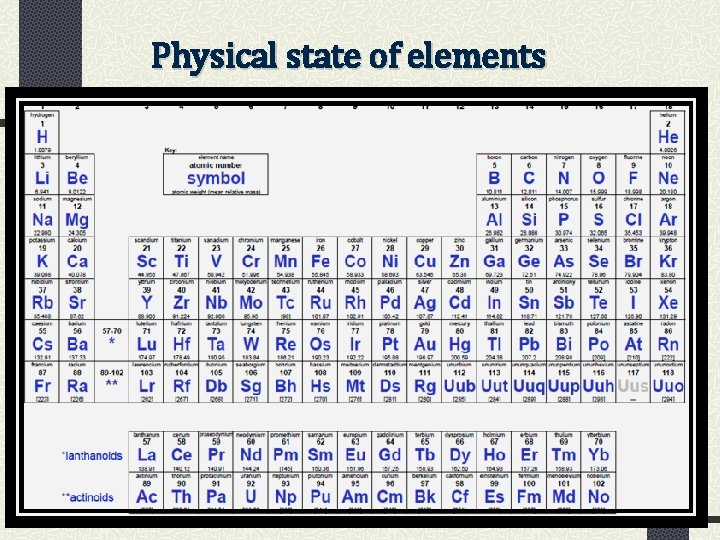Physical state of elements 11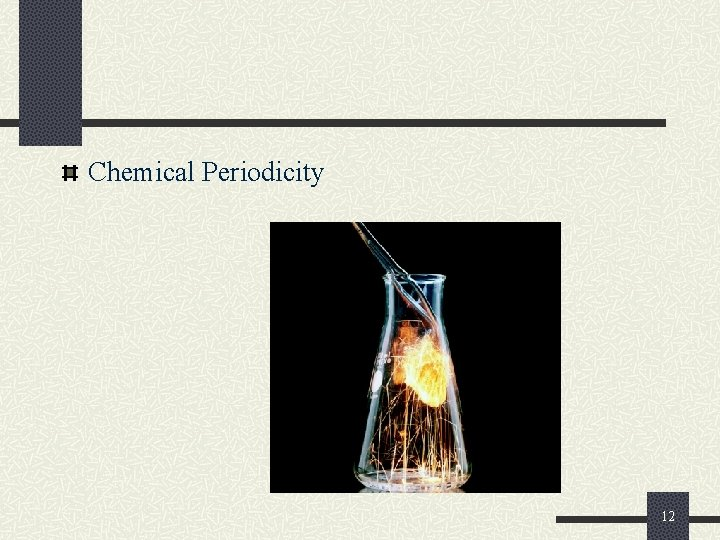Chemical Periodicity 12Periodic Properties of the Elements Atomic Radii Atomic radii describes the relative sizes of atoms. It is understood as the distance from the nucleus to the outermost occupied energy level. Atomic radii increase within a column going from the top to the bottom of the periodic table. Atomic radii decrease within a row going from left to right on the periodic table. 13Atomic Radii Example: Arrange these elements based on their atomic radii. n Se, S, O, Te 14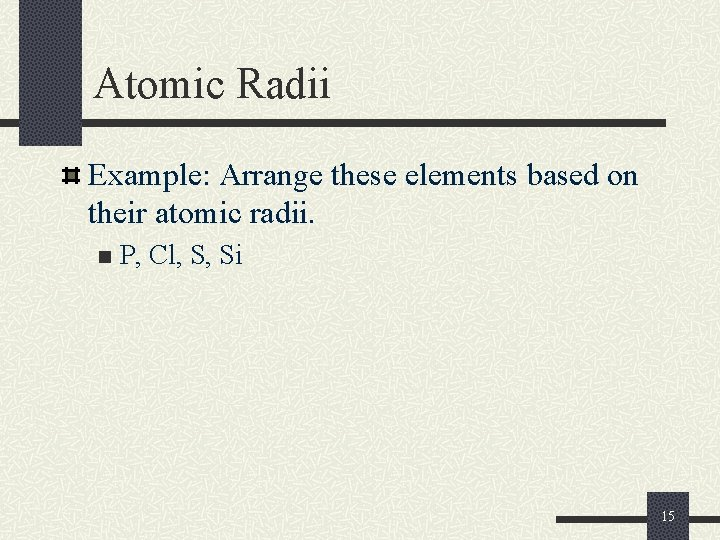Atomic Radii Example: Arrange these elements based on their atomic radii. n P, Cl, S, Si 15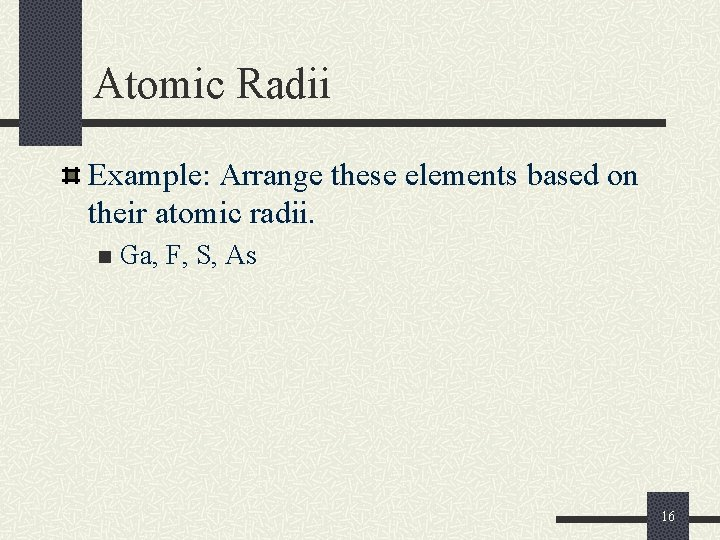Atomic Radii Example: Arrange these elements based on their atomic radii. n Ga, F, S, As 16Ionization Energy First ionization energy (IE 1) n The minimum amount of energy required to remove the most loosely bound electron from an isolated gaseous atom to form a 1+ ion. Symbolically: Atom(g) + energy ion+(g) + e- Mg(g) + 738 k. J/mol Mg+ + e- 17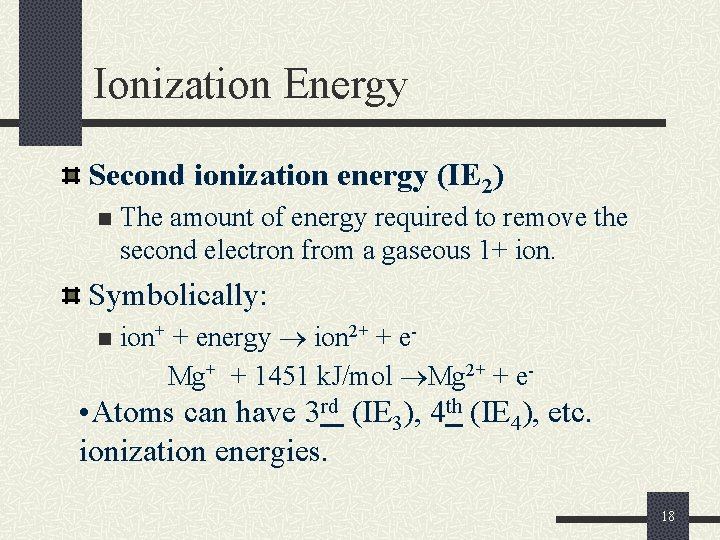Ionization Energy Second ionization energy (IE 2) n The amount of energy required to remove the second electron from a gaseous 1+ ion. Symbolically: n ion+ + energy ion 2+ + e. Mg+ + 1451 k. J/mol Mg 2+ + e- • Atoms can have 3 rd (IE 3), 4 th (IE 4), etc. ionization energies. 18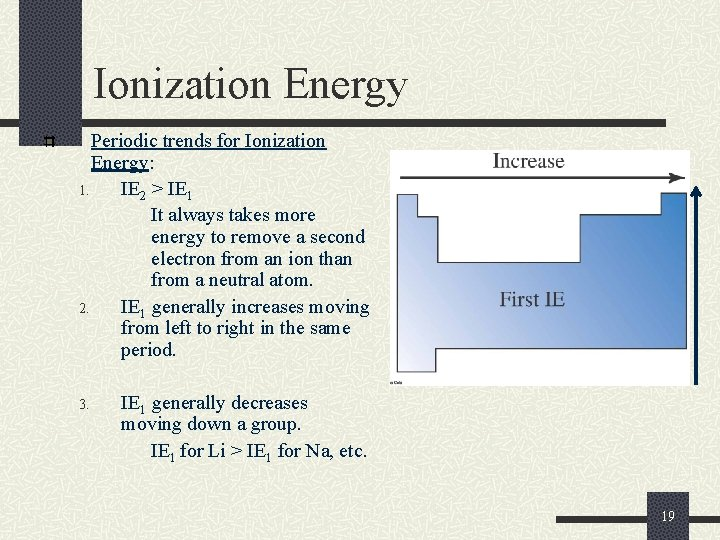Ionization Energy Periodic trends for Ionization Energy: 1. IE 2 > IE 1 It always takes more energy to remove a second electron from an ion than from a neutral atom. 2. IE 1 generally increases moving from left to right in the same period. 3. IE 1 generally decreases moving down a group. IE 1 for Li > IE 1 for Na, etc. 19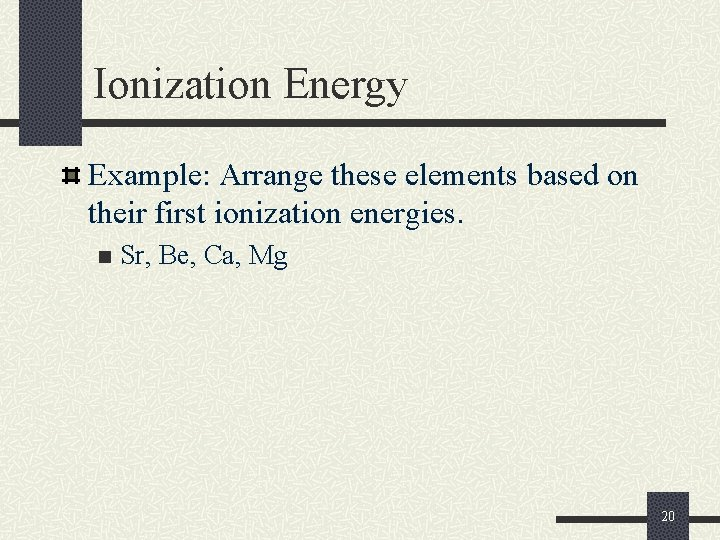Ionization Energy Example: Arrange these elements based on their first ionization energies. n Sr, Be, Ca, Mg 20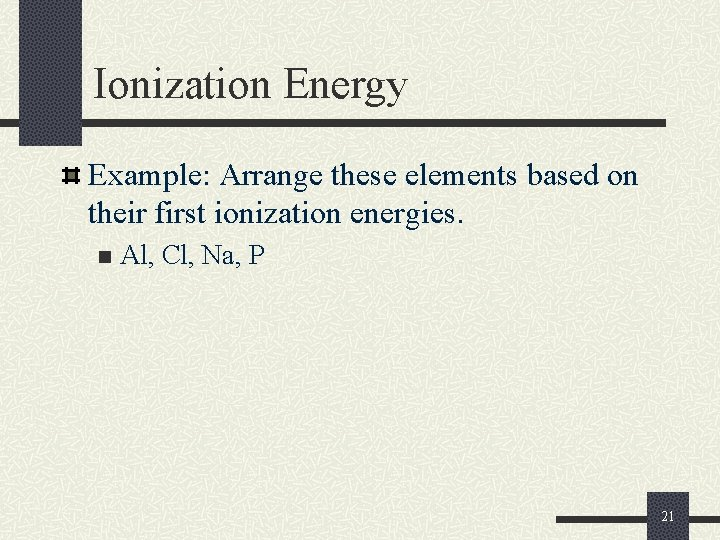Ionization Energy Example: Arrange these elements based on their first ionization energies. n Al, Cl, Na, P 21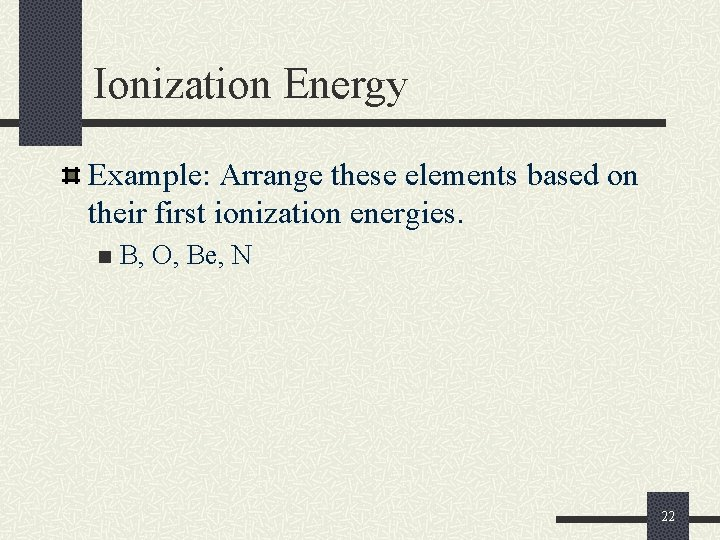Ionization Energy Example: Arrange these elements based on their first ionization energies. n B, O, Be, N 22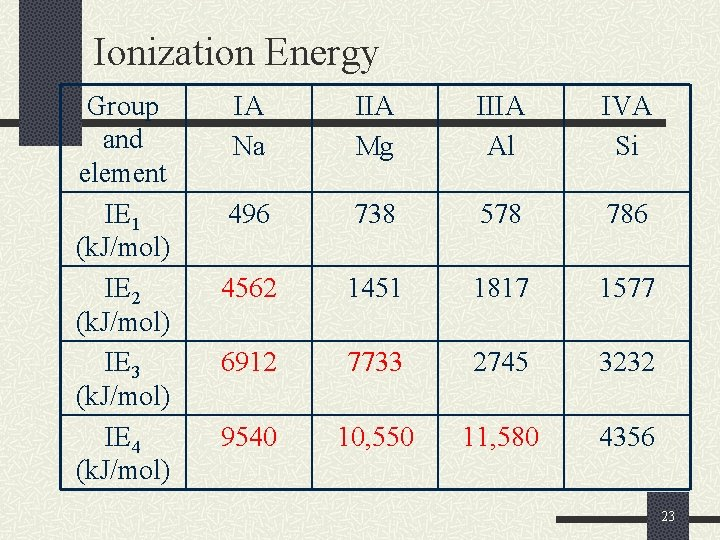Ionization Energy Group and element IE 1 (k. J/mol) IE 2 (k. J/mol) IE 3 (k. J/mol) IE 4 (k. J/mol) IA Na IIA Mg IIIA Al IVA Si 496 738 578 786 4562 1451 1817 1577 6912 7733 2745 3232 9540 10, 550 11, 580 4356 23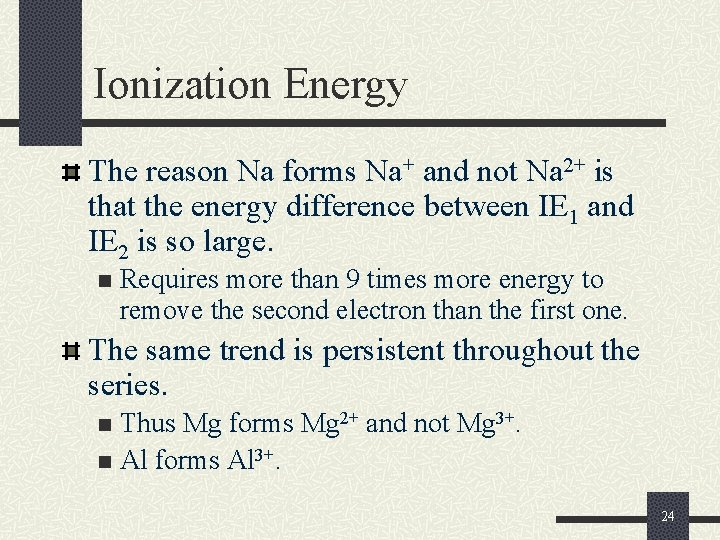Ionization Energy The reason Na forms Na+ and not Na 2+ is that the energy difference between IE 1 and IE 2 is so large. n Requires more than 9 times more energy to remove the second electron than the first one. The same trend is persistent throughout the series. Thus Mg forms Mg 2+ and not Mg 3+. n Al forms Al 3+. n 24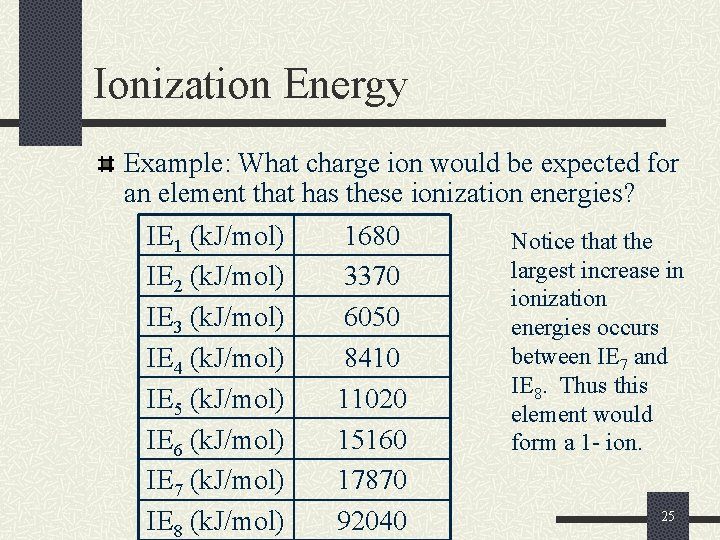Ionization Energy Example: What charge ion would be expected for an element that has these ionization energies? IE 1 (k. J/mol) IE 2 (k. J/mol) IE 3 (k. J/mol) IE 4 (k. J/mol) IE 5 (k. J/mol) IE 6 (k. J/mol) IE 7 (k. J/mol) IE 8 (k. J/mol) 1680 3370 6050 8410 11020 15160 17870 92040 Notice that the largest increase in ionization energies occurs between IE 7 and IE 8. Thus this element would form a 1 - ion. 25Ionization Energy Example: What charge ion would be expected for an element that has these ionization energies? IE 1 (k. J/mol) IE 2 (k. J/mol) IE 3 (k. J/mol) IE 4 (k. J/mol) IE 5 (k. J/mol) IE 6 (k. J/mol) IE 7 (k. J/mol) IE 8 (k. J/mol) 1680 3370 11586 12410 13020 15160 17870 18040 Notice that the largest increase in ionization energies occurs between IE 2 and IE 3. Thus this element would form a 2+ ion. 26Ionization Energy Example: What charge ion would be expected for an element that has these ionization energies? IE 1 (k. J/mol) IE 2 (k. J/mol) IE 3 (k. J/mol) IE 4 (k. J/mol) IE 5 (k. J/mol) IE 6 (k. J/mol) IE 7 (k. J/mol) IE 8 (k. J/mol) 1680 3370 4586 5410 6020 7160 17870 18040 Notice that the largest increase in ionization energies occurs between IE 6 and IE 7. Thus this element would form a 2 - ion. 27Ionic Radii Cations (positive ions) are always smaller than their respective neutral atoms. Element Li Be Atomic Radius (Å) Ion 1. 52 1. 12 Li+ Be 2+ Ionic Radius (Å) 0. 90 0. 59 28Ionic Radii Cations (positive ions) are always smaller than their respective neutral atoms. Element Na Mg Al Atomic Radius (Å) 1. 86 1. 60 1. 43 Ion Na+ Mg 2+ Al 3+ Ionic Radius (Å) 1. 16 0. 85 0. 68 29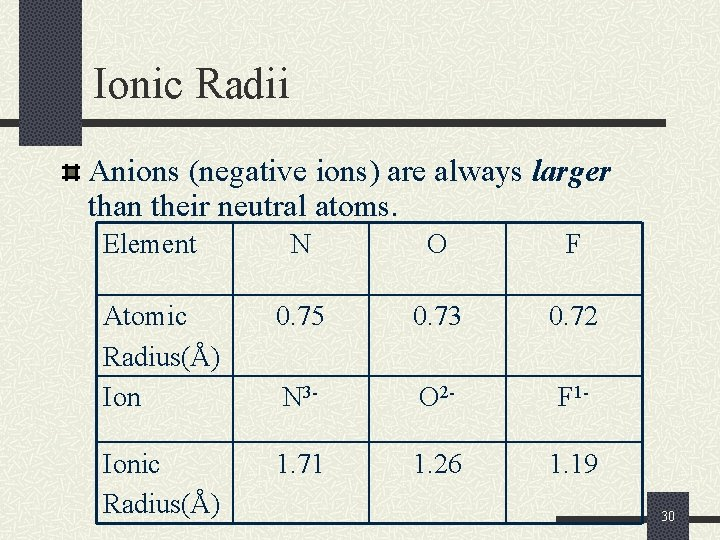Ionic Radii Anions (negative ions) are always larger than their neutral atoms. Element N O F Atomic Radius(Å) Ion 0. 75 0. 73 0. 72 N 3 - O 2 - F 1 - Ionic Radius(Å) 1. 71 1. 26 1. 19 30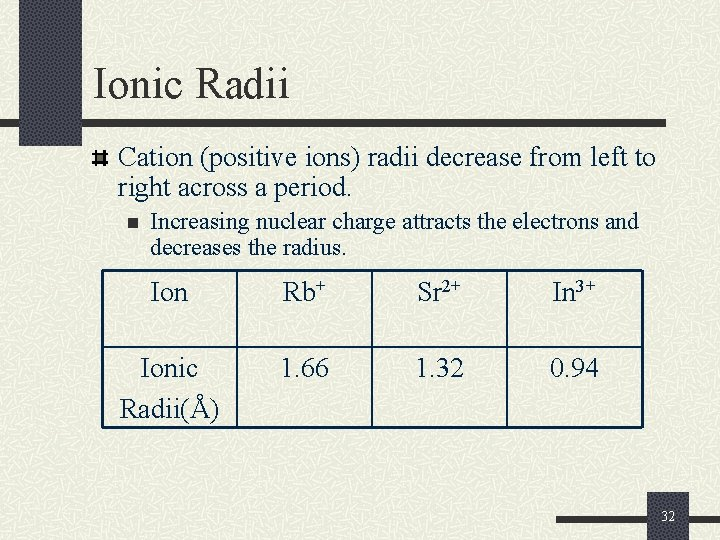Ionic Radii Cation (positive ions) radii decrease from left to right across a period. n Increasing nuclear charge attracts the electrons and decreases the radius. Ion Rb+ Sr 2+ In 3+ Ionic Radii(Å) 1. 66 1. 32 0. 94 32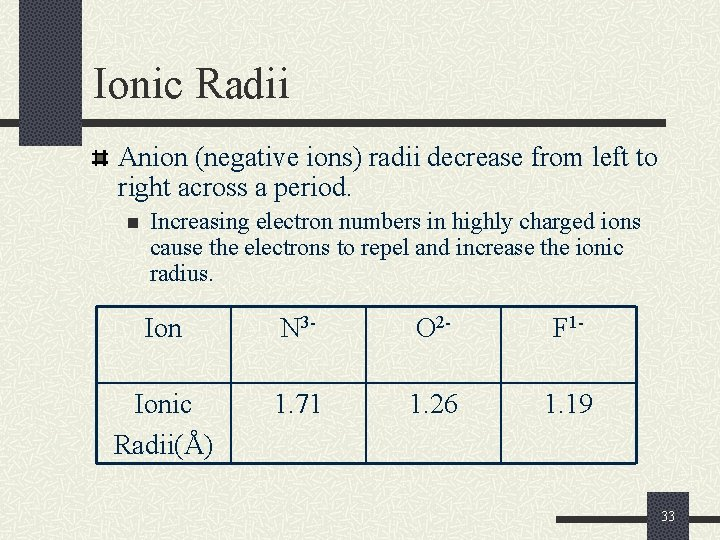Ionic Radii Anion (negative ions) radii decrease from left to right across a period. n Increasing electron numbers in highly charged ions cause the electrons to repel and increase the ionic radius. Ion N 3 - O 2 - F 1 - Ionic Radii(Å) 1. 71 1. 26 1. 19 33Ionic Radii Example: Arrange these elements based on their ionic radii. n Ga, K, Ca 34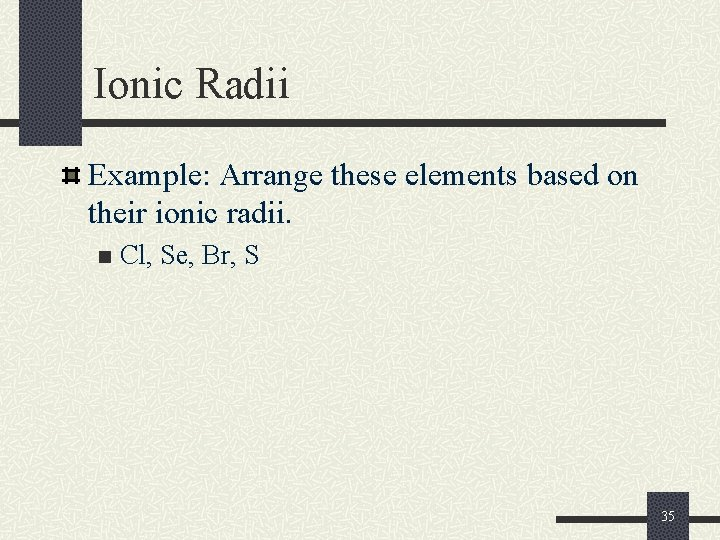Ionic Radii Example: Arrange these elements based on their ionic radii. n Cl, Se, Br, S 35Electronegativity is a measure of the relative tendency of an atom to attract electrons to itself when chemically combined with another element. n Electronegativity is measured on the Pauling scale. n Fluorine is the most electronegative element. n Cesium and francium are the least electronegative elements. For the representative elements, electronegativities usually increase from left to right across periods and decrease from top to bottom within groups. 36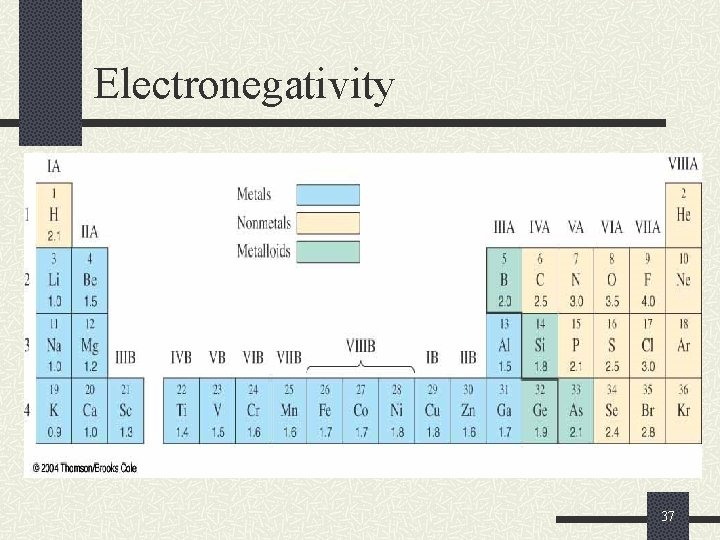Electronegativity 37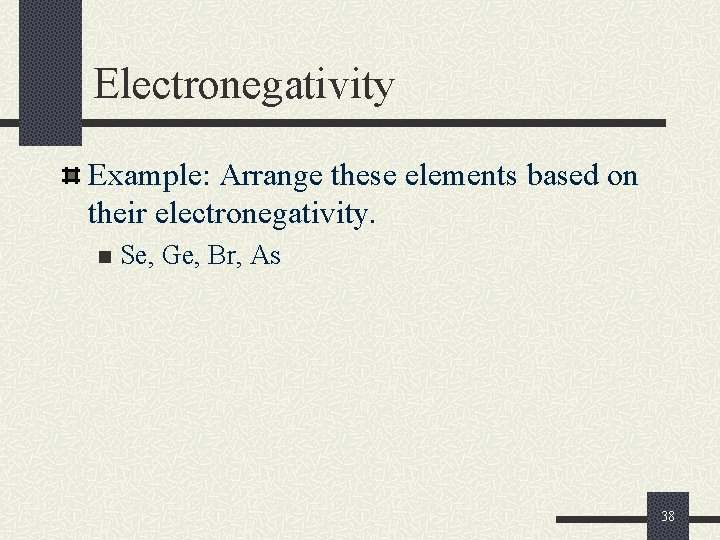Electronegativity Example: Arrange these elements based on their electronegativity. n Se, Ge, Br, As 38Electronegativity Example: Arrange these elements based on their electronegativity. n Be, Mg, Ca, Ba 39See animation for Summary of Trends in the Periodic Table http: //www. learnerstv. com/animation. php? ani=56&cat=chemistry 40